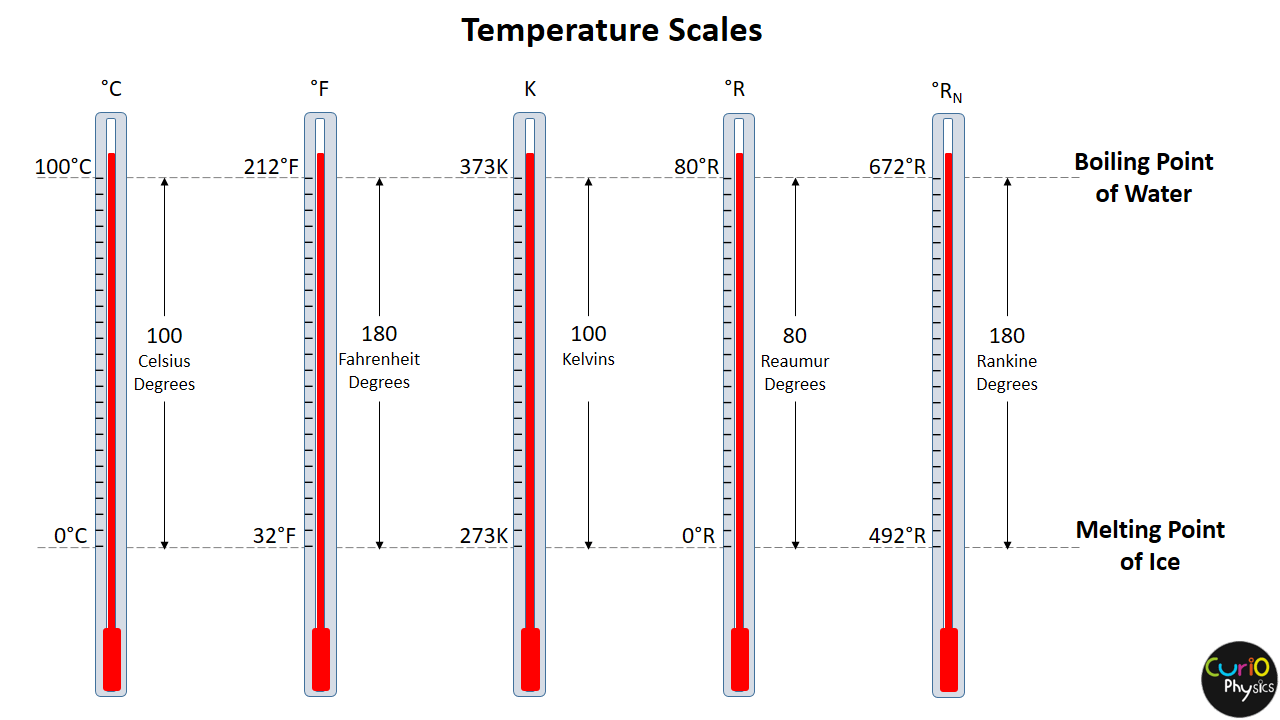# Thermal Expansion

Most materials expand when their temperatures increase. This phenomenon is  known as thermal expansion and it has an important role in numerous engineering applications. For example, thermal-expansion joints, are included in buildings, concrete highways, railroad tracks, brick walls, and bridges to compensate for dimensional changes that occur as the temperature changes.

##### Reason of Thermal expansion :-

Thermal expansion is a consequence of the change in the average separation between the atoms in an object. At ordinary temperatures, the atoms in a solid oscillate about their equilibrium positions with a frequency of approximately 1013 Hz. The average spacing between the atoms is about 10-10 m. As the temperature of the solid increases, the atoms oscillate with greater amplitudes; as a result, the average separation between them increases. Consequently, the object expands.

##### Types of thermal expansion :-

Thermal Expansion is of three types :-

1. Linear Expansion
2. Area / areal Expansion
3. Volume / volumetric expansion
###### (1) Linear Expansion :-

Suppose a rod of some material has a length L0 at some initial temperature T0. Now if the temperature of the rod increases by ΔT, the length of the rod changes by ΔL, then experiments show that [if ΔT is not too large (say, less than 100ºC or so)] :-

(i) ΔL is directly proportional to ΔT

i.e.      ΔL ∝ ΔT     ……….(1)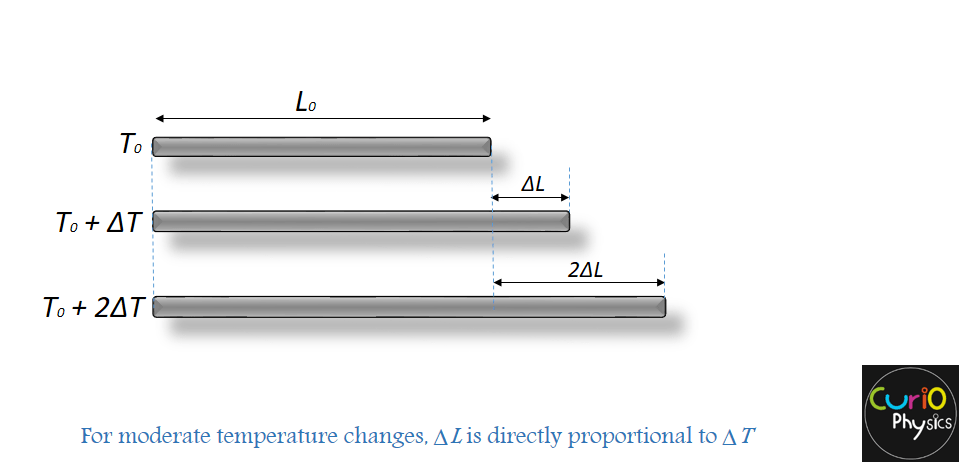If two rods are made of the same material and have the same temperature change, but one is twice as long as the other, then the change in its length is also twice as great. Therefore

(ii) ΔL must be directly proportional to L0

i.e.      ΔL ∝ L0     ……….(2)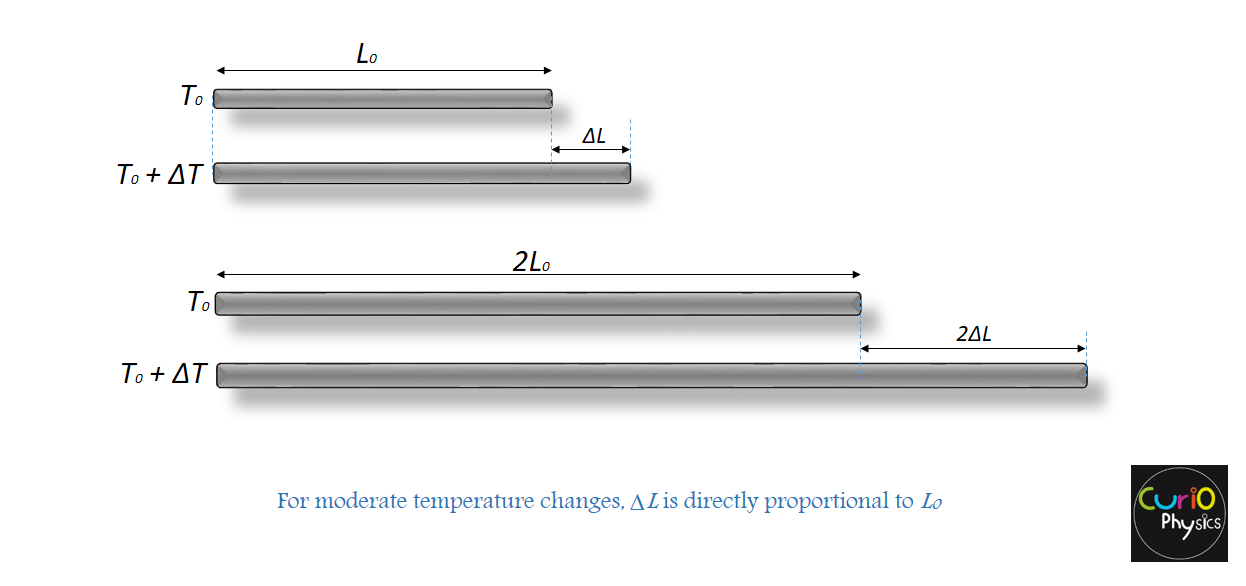Now using equations (1) and (2) we get:-

ΔL ∝ L0ΔT

introducing a proportionality constant α (which is different for different materials), we may express above relation in an equation:-

ΔL = αL0ΔT     ……….(3)

If a rod has length L0 at temperature T0 then its length L at a temperature  T = T0+ΔT  is

L = L0 + ΔL = L0 + αL0ΔT

or

L = L0 (1 + αΔT )     ……….(4)

The constant α which describes the thermal expansion properties of a particular material, is called the coefficient of linear expansion. The units of α are K-1 or ºC-1.
This is because no matter what unit of temperature you are taking ( K or ºC) , the temperature interval(change in temperature) is the same in the Kelvin and Celsius scales.

From ΔL = αL0ΔT , we get

α = ΔL / L0ΔT

From above equation….

Definition of α :- The coefficient of liner expansion α may be defined as change in length(ΔL), per unit original length(L0), per degree Celsius (or per Kelvin) rise in temperature(ΔT).

Note :- It may be helpful to think of thermal expansion as an effective magnification of an object. For example, when a metal washer is heated all dimensions, including the radius of the hole, increase according to
Equation (4).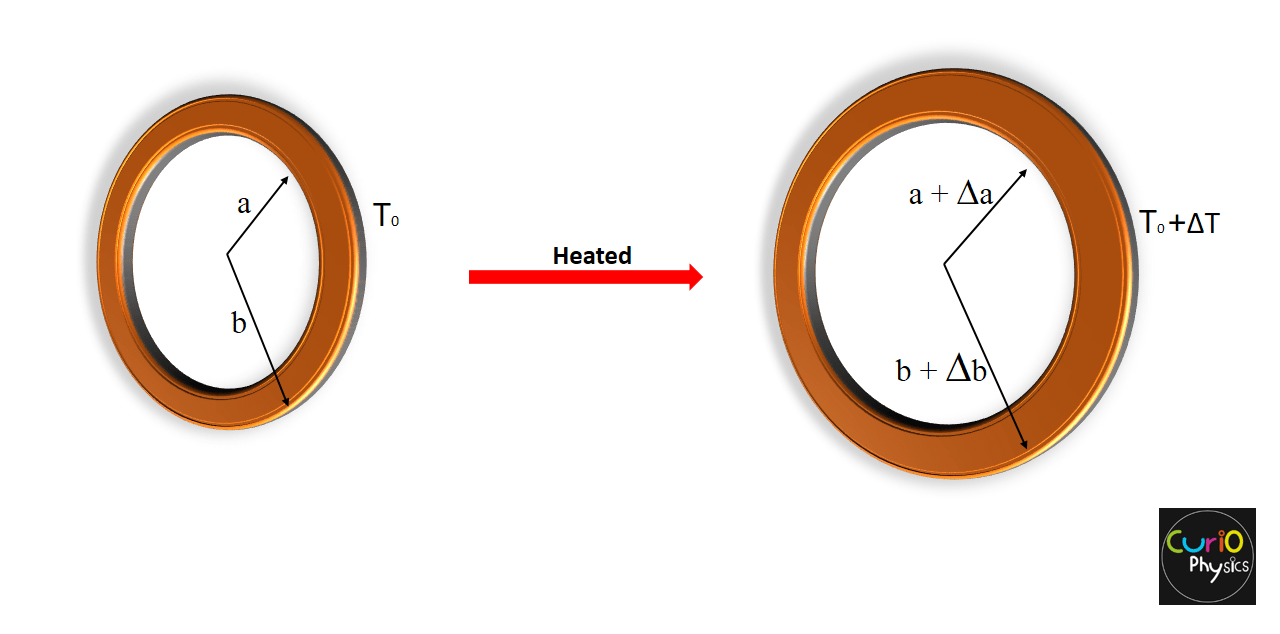Notice that this is equivalent to saying that a cavity in a piece of material expands in the same way as if the cavity were filled with the material.

Because the linear dimensions of an object change with temperature, it follows that surface area and volume change as well :-

###### (2) area / areal Expansion :-

Suppose a sheet of some material has an area A0 at some initial temperature T0. Now if the temperature of the sheet increases by ΔT, the area of the sheet changes by ΔA.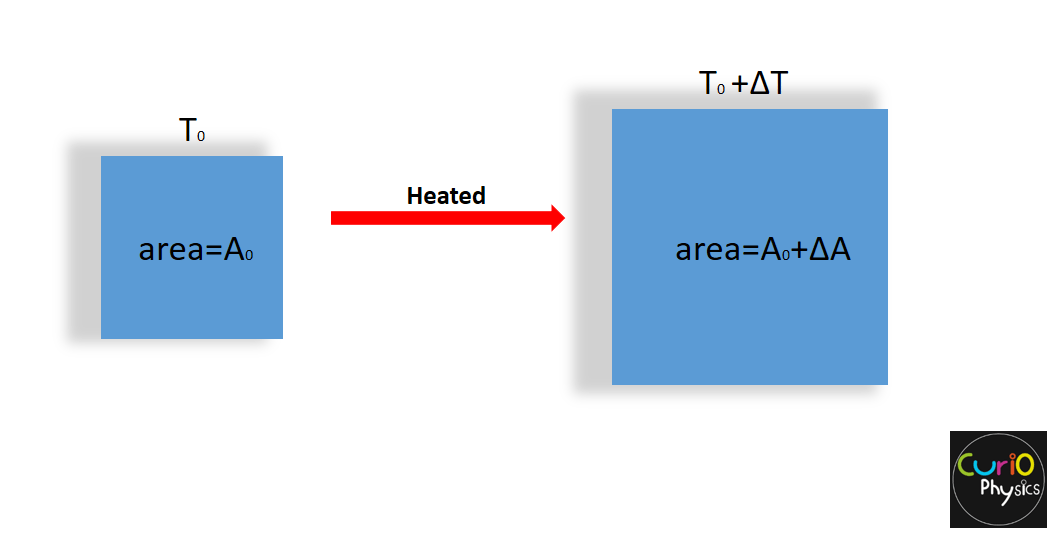Experiments show that [if ΔT is not too large (say, less than 100ºC or so)] :-

(i) ΔA is directly proportional to ΔT

i.e.      ΔA ∝ ΔT     ……….(5)

and

(ii) ΔA is also directly proportional to A0

i.e.      ΔA ∝ A0     ……….(6)

From (5) and (6)

ΔA ∝ A0ΔT

removing proportionality sign….

ΔA =βA0ΔT     ……….(7)

If the sheet has area A0 at temperature T0 then its area A at a temperature  T = T0+ΔT  is

A = A0 + ΔA = A0 + β A0ΔT

or

A = A0 (1 + βΔT )……….(8)

The constant β which describes the thermal expansion properties of a particular material, is called the coefficient of areal/area expansion. The units of β are K-1 or ºC-1.

From ΔA = βA0ΔT , we get

β = ΔA / A0ΔT

From above equation….

Definition of β :- The coefficient of areal expansion β may be defined as change in area(ΔA), per unit original area(A0), per degree Celsius (or per Kelvin) rise in temperature(ΔT).

###### (3) Volumetric / volume Expansion :-

Suppose a cube of some material has a volume V0 at some initial temperature T0. Now if the temperature of the cube increases by ΔT, the volume of the cube changes by ΔV.Experiments show that [if ΔT is not too large (say, less than 100ºC or so)] :-

(i) ΔV is directly proportional to ΔT

i.e.      ΔV ∝ ΔT     ……….(9)

and

(ii) ΔV must be directly proportional to V0

i.e.      ΔV ∝ V0     ……….(10)

From (9) and (10)

ΔV ∝ V0ΔT

removing proportionality sign….

ΔV =γV0ΔT     ……….(11)

If the cube has volume V0 at temperature T0 then its volume V at a temperature  T = T0+ΔT  is

V = V0 + ΔV = V0 + γV0ΔT

or

V = V0(1 + γΔT )……….(12)

The constant γ which describes the thermal expansion properties of a particular material, is called the coefficient of volumetric/volume expansion. The units of γ are K-1 or ºC-1.

From ΔV = γV0ΔT , we get

γ = ΔV / V0ΔT

From above equation….

Definition of γ :- The coefficient of volumetric expansion γ may be defined as change in volume(ΔV), per unit original volume(V0), per degree Celsius (or per Kelvin) rise in temperature(ΔT).